Скачать презентацию Magnetism III Magnetic Ordering Physics 355 So

5abf0ca0aa5d54aa1236bb17bd7997ec.ppt

• Количество слайдов: 27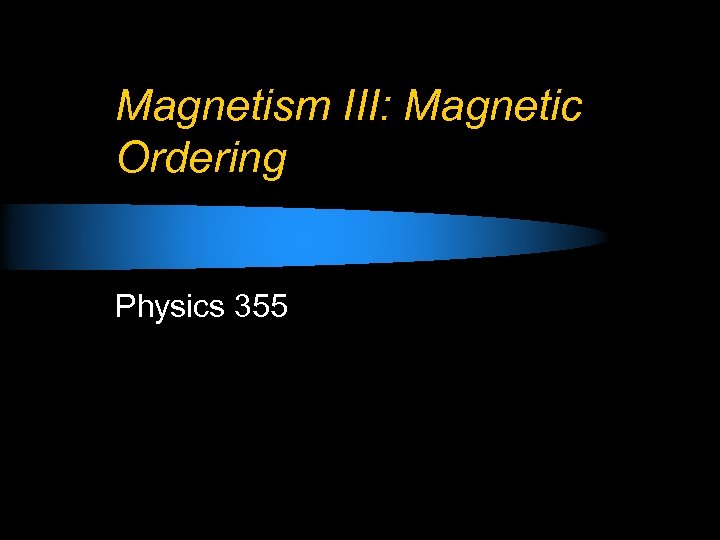Magnetism III: Magnetic Ordering Physics 355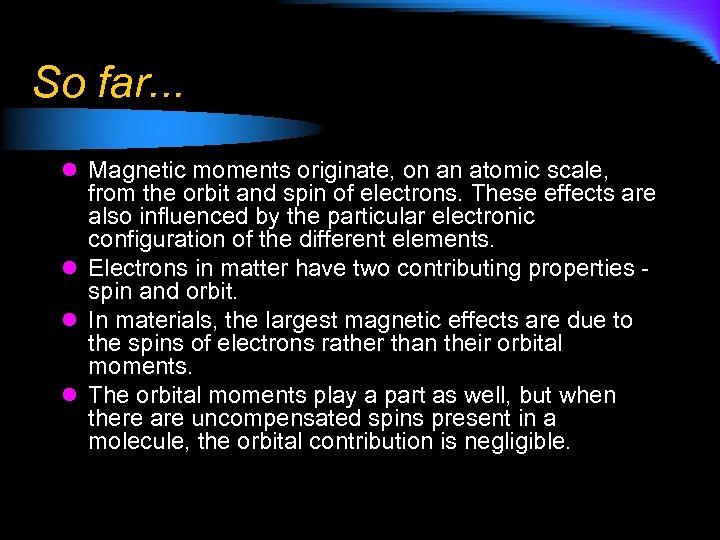So far. . . l Magnetic moments originate, on an atomic scale, from the orbit and spin of electrons. These effects are also influenced by the particular electronic configuration of the different elements. l Electrons in matter have two contributing properties - spin and orbit. l In materials, the largest magnetic effects are due to the spins of electrons rather than their orbital moments. l The orbital moments play a part as well, but when there are uncompensated spins present in a molecule, the orbital contribution is negligible.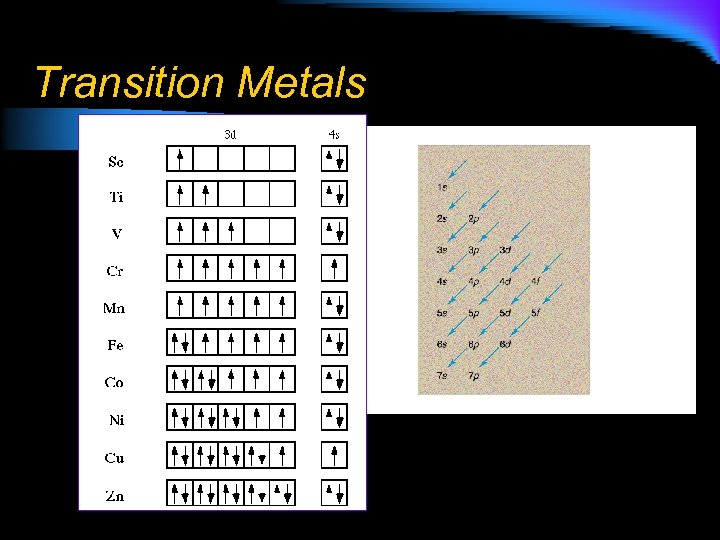Transition Metals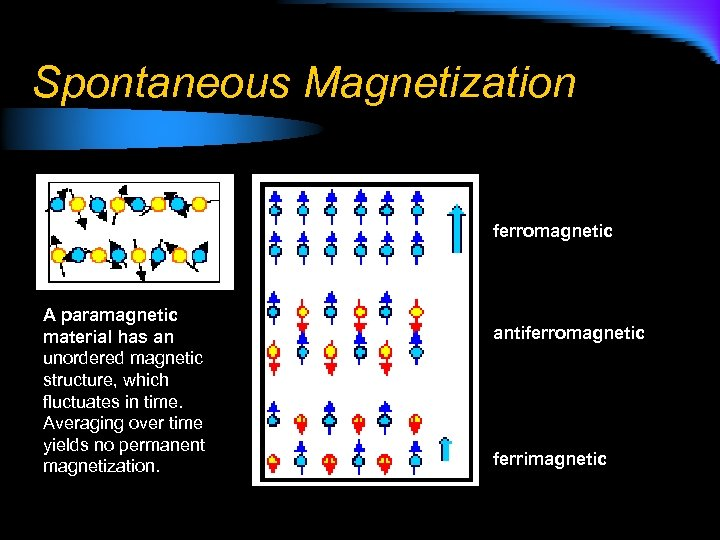Spontaneous Magnetization ferromagnetic A paramagnetic material has an unordered magnetic structure, which fluctuates in time. Averaging over time yields no permanent magnetization. antiferromagnetic ferrimagnetic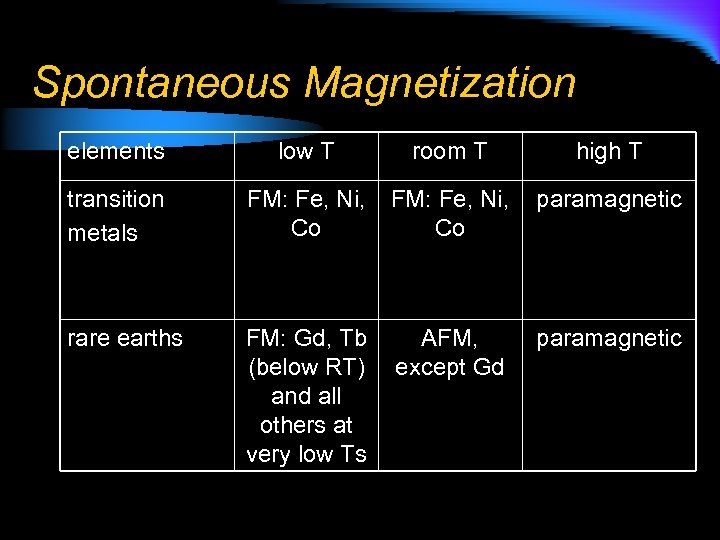Spontaneous Magnetization elements low T room T high T transition metals FM: Fe, Ni, paramagnetic Co Co rare earths FM: Gd, Tb AFM, (below RT) except Gd and all others at very low Ts paramagnetic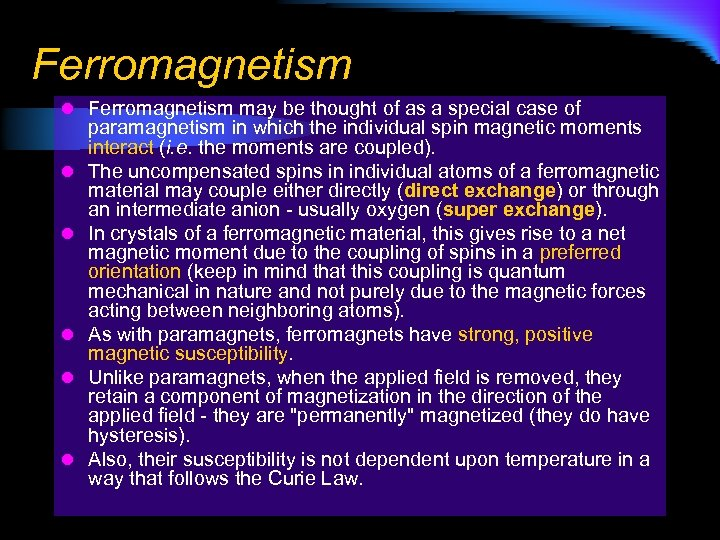Ferromagnetism l Ferromagnetism may be thought of as a special case of l l l paramagnetism in which the individual spin magnetic moments interact (i. e. the moments are coupled). The uncompensated spins in individual atoms of a ferromagnetic material may couple either directly (direct exchange) or through an intermediate anion - usually oxygen (super exchange). In crystals of a ferromagnetic material, this gives rise to a net magnetic moment due to the coupling of spins in a preferred orientation (keep in mind that this coupling is quantum mechanical in nature and not purely due to the magnetic forces acting between neighboring atoms). As with paramagnets, ferromagnets have strong, positive magnetic susceptibility. Unlike paramagnets, when the applied field is removed, they retain a component of magnetization in the direction of the applied field - they are "permanently" magnetized (they do have hysteresis). Also, their susceptibility is not dependent upon temperature in a way that follows the Curie Law.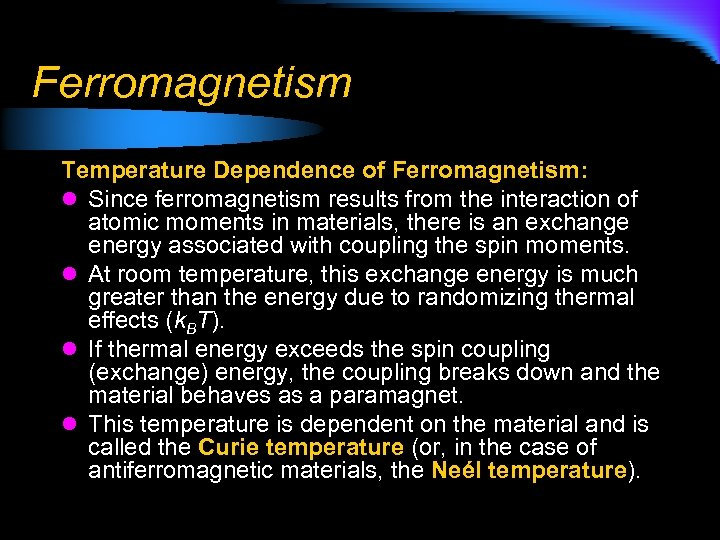Ferromagnetism Temperature Dependence of Ferromagnetism: l Since ferromagnetism results from the interaction of atomic moments in materials, there is an exchange energy associated with coupling the spin moments. l At room temperature, this exchange energy is much greater than the energy due to randomizing thermal effects (k. BT). l If thermal energy exceeds the spin coupling (exchange) energy, the coupling breaks down and the material behaves as a paramagnet. l This temperature is dependent on the material and is called the Curie temperature (or, in the case of antiferromagnetic materials, the Neél temperature).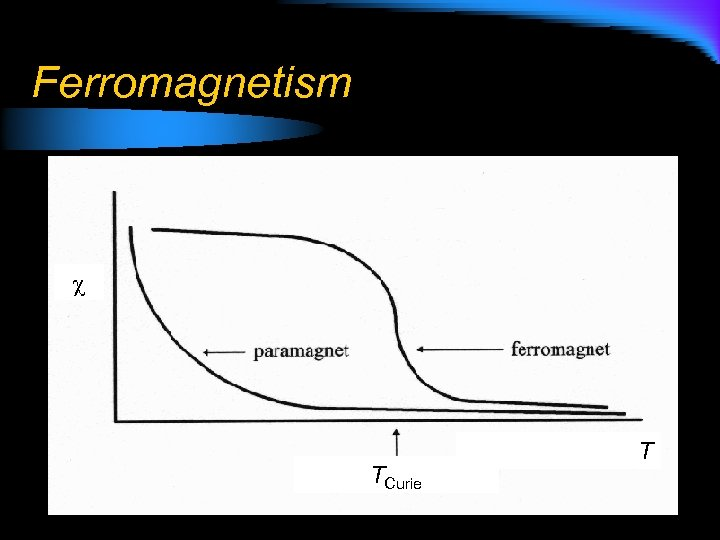Ferromagnetism TCurie T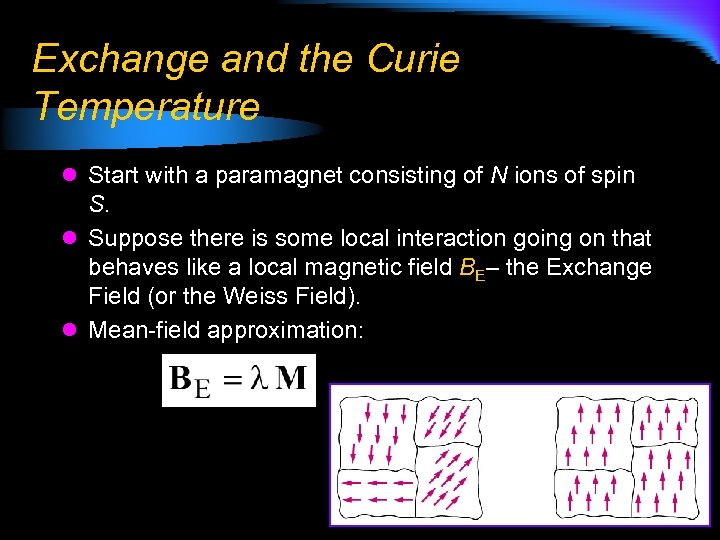Exchange and the Curie Temperature l Start with a paramagnet consisting of N ions of spin S. l Suppose there is some local interaction going on that behaves like a local magnetic field BE– the Exchange Field (or the Weiss Field). l Mean-field approximation: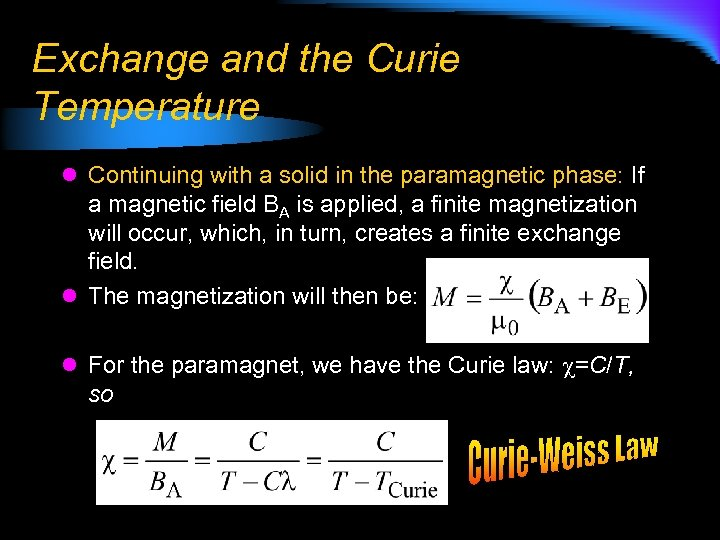Exchange and the Curie Temperature l Continuing with a solid in the paramagnetic phase: If a magnetic field BA is applied, a finite magnetization will occur, which, in turn, creates a finite exchange field. l The magnetization will then be: l For the paramagnet, we have the Curie law: =C/T, so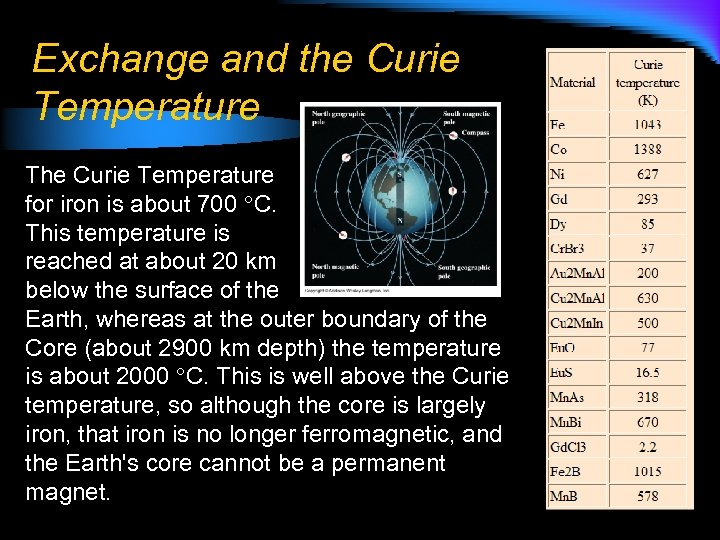Exchange and the Curie Temperature The Curie Temperature for iron is about 700 C. This temperature is reached at about 20 km below the surface of the Earth, whereas at the outer boundary of the Core (about 2900 km depth) the temperature is about 2000 C. This is well above the Curie temperature, so although the core is largely iron, that iron is no longer ferromagnetic, and the Earth's core cannot be a permanent magnet.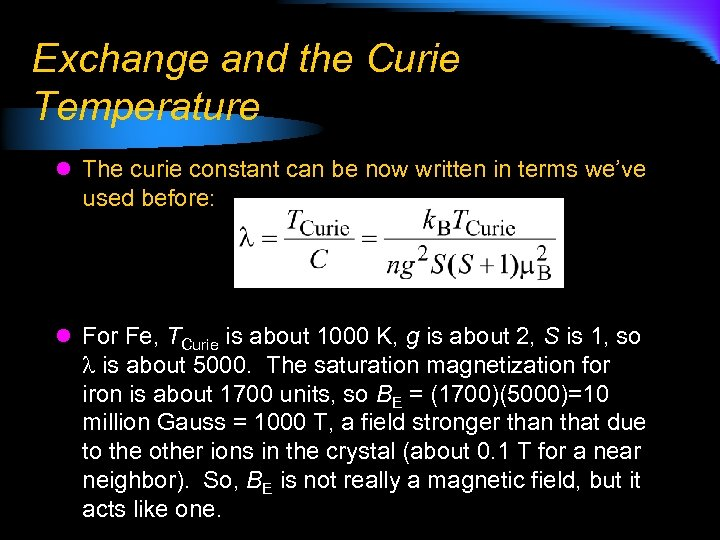Exchange and the Curie Temperature l The curie constant can be now written in terms we’ve used before: l For Fe, TCurie is about 1000 K, g is about 2, S is 1, so is about 5000. The saturation magnetization for iron is about 1700 units, so BE = (1700)(5000)=10 million Gauss = 1000 T, a field stronger than that due to the other ions in the crystal (about 0. 1 T for a near neighbor). So, BE is not really a magnetic field, but it acts like one.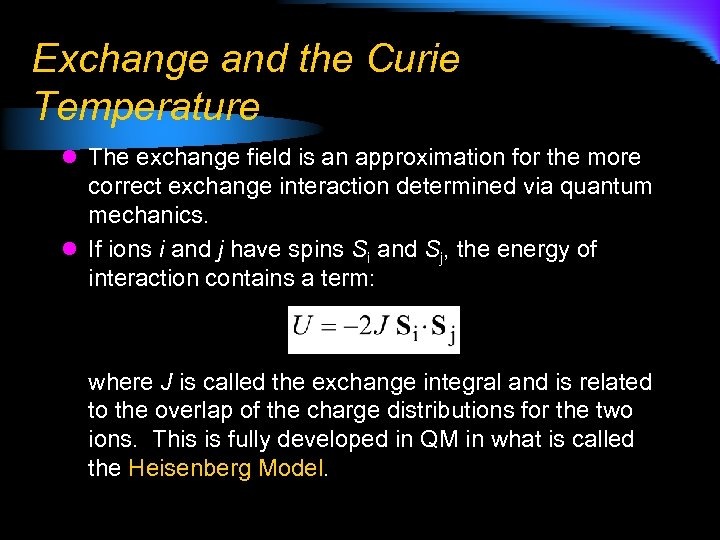Exchange and the Curie Temperature l The exchange field is an approximation for the more correct exchange interaction determined via quantum mechanics. l If ions i and j have spins Si and Sj, the energy of interaction contains a term: where J is called the exchange integral and is related to the overlap of the charge distributions for the two ions. This is fully developed in QM in what is called the Heisenberg Model.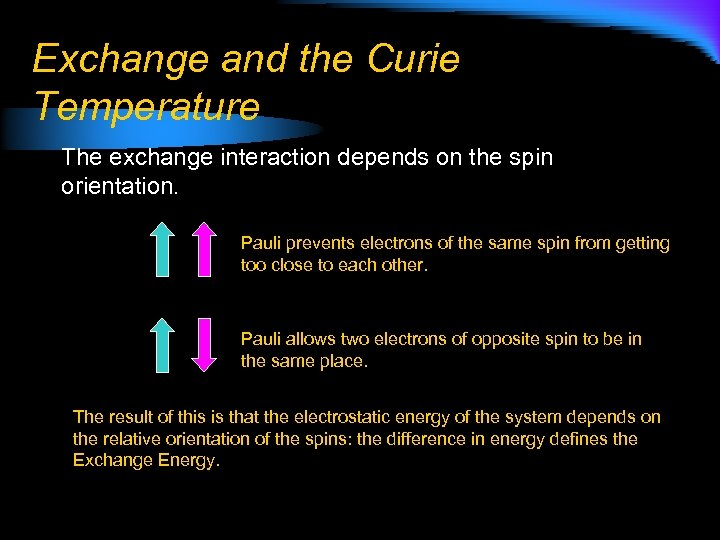Exchange and the Curie Temperature The exchange interaction depends on the spin orientation. Pauli prevents electrons of the same spin from getting too close to each other. Pauli allows two electrons of opposite spin to be in the same place. The result of this is that the electrostatic energy of the system depends on the relative orientation of the spins: the difference in energy defines the Exchange Energy.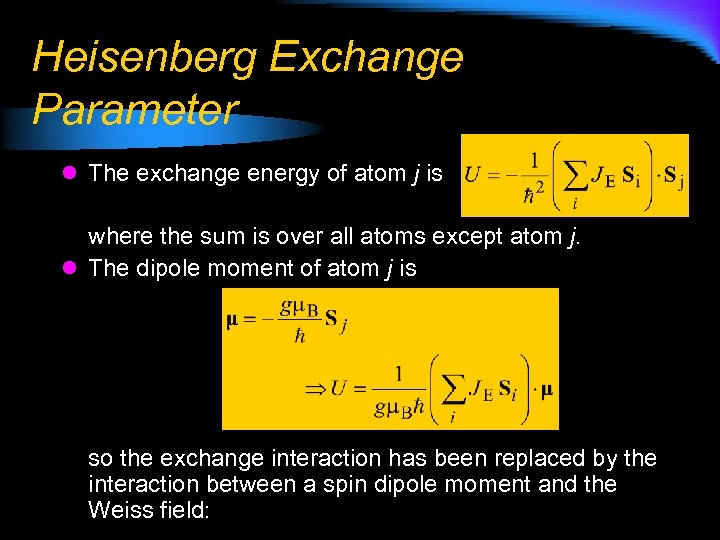Heisenberg Exchange Parameter l The exchange energy of atom j is where the sum is over all atoms except atom j. l The dipole moment of atom j is so the exchange interaction has been replaced by the interaction between a spin dipole moment and the Weiss field: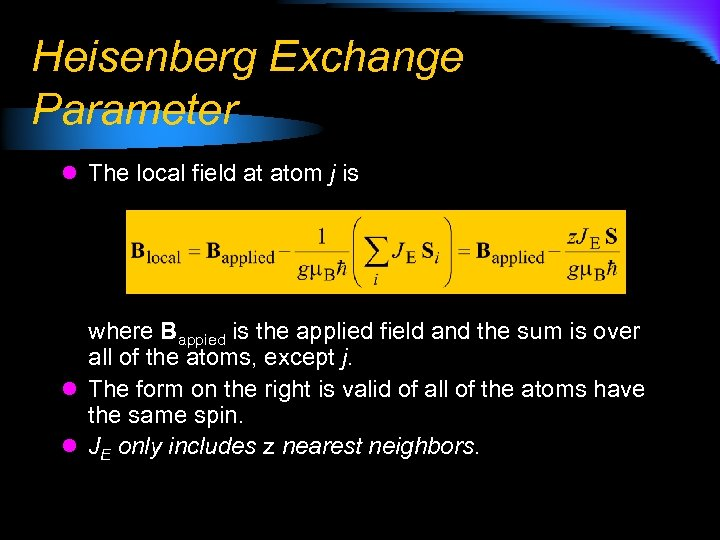Heisenberg Exchange Parameter l The local field at atom j is where Bappied is the applied field and the sum is over all of the atoms, except j. l The form on the right is valid of all of the atoms have the same spin. l JE only includes z nearest neighbors.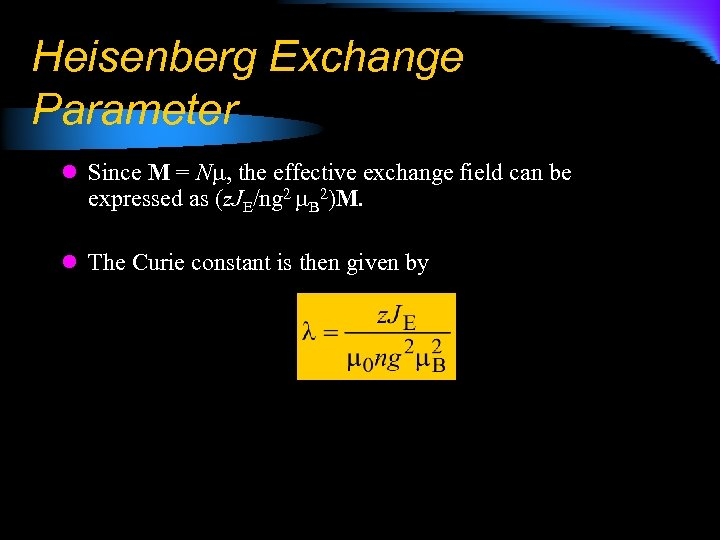Heisenberg Exchange Parameter l Since M = N , the effective exchange field can be expressed as (z. JE/ng 2 B 2)M. l The Curie constant is then given by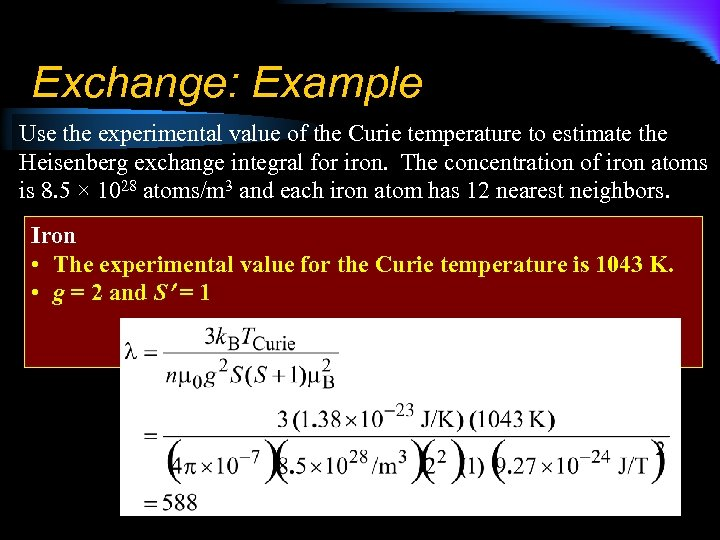Exchange: Example Use the experimental value of the Curie temperature to estimate the Heisenberg exchange integral for iron. The concentration of iron atoms is 8. 5 × 1028 atoms/m 3 and each iron atom has 12 nearest neighbors. Iron • The experimental value for the Curie temperature is 1043 K. • g = 2 and S = 1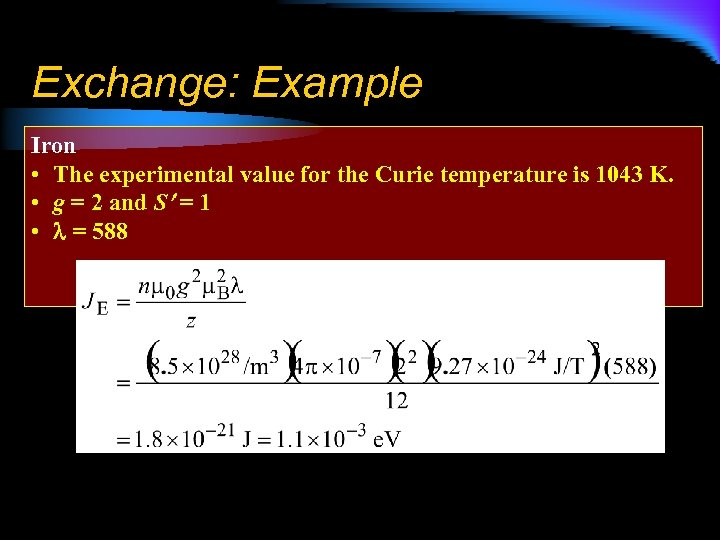Exchange: Example Iron • The experimental value for the Curie temperature is 1043 K. • g = 2 and S = 1 • = 588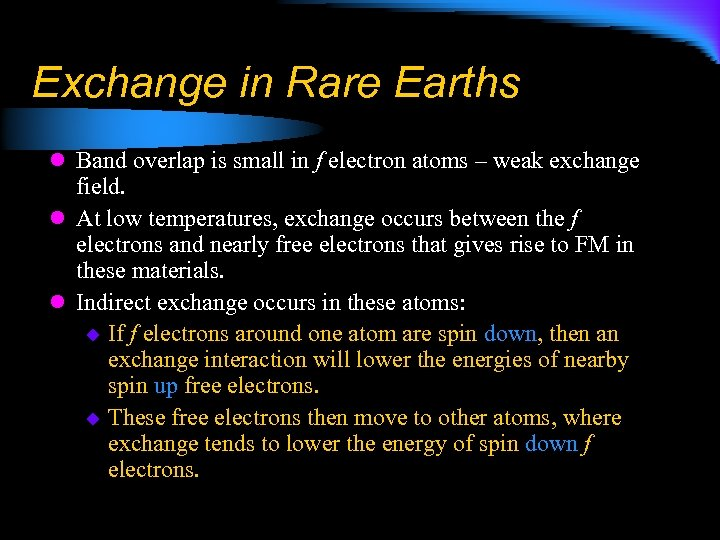Exchange in Rare Earths l Band overlap is small in f electron atoms – weak exchange field. l At low temperatures, exchange occurs between the f electrons and nearly free electrons that gives rise to FM in these materials. l Indirect exchange occurs in these atoms: u If f electrons around one atom are spin down, then an exchange interaction will lower the energies of nearby spin up free electrons. u These free electrons then move to other atoms, where exchange tends to lower the energy of spin down f electrons.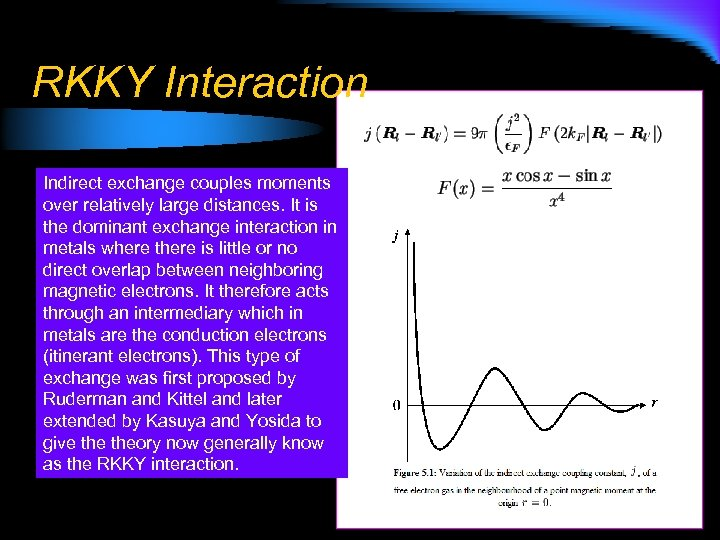RKKY Interaction Indirect exchange couples moments over relatively large distances. It is the dominant exchange interaction in metals where there is little or no direct overlap between neighboring magnetic electrons. It therefore acts through an intermediary which in metals are the conduction electrons (itinerant electrons). This type of exchange was first proposed by Ruderman and Kittel and later extended by Kasuya and Yosida to give theory now generally know as the RKKY interaction.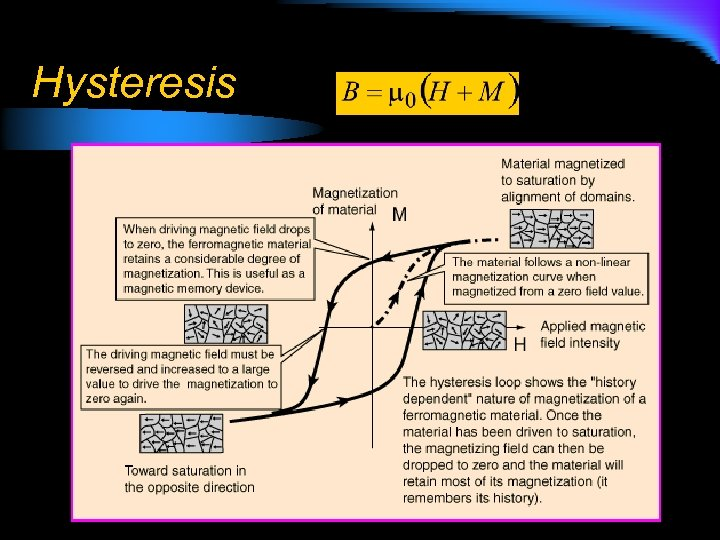Hysteresis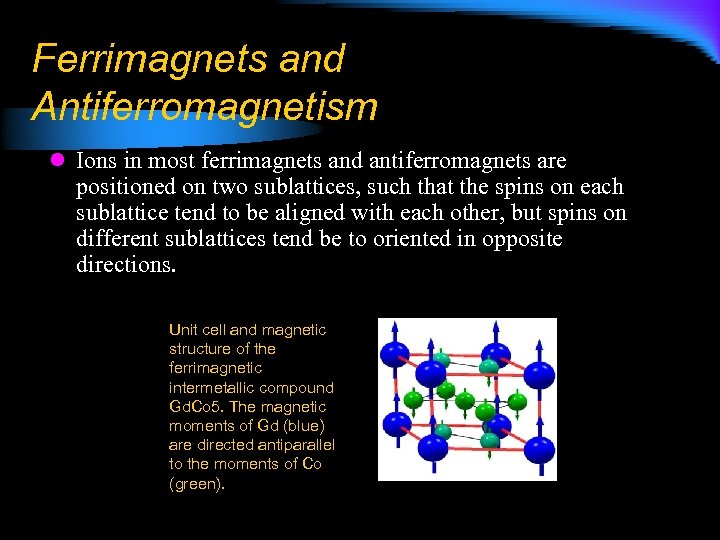Ferrimagnets and Antiferromagnetism l Ions in most ferrimagnets and antiferromagnets are positioned on two sublattices, such that the spins on each sublattice tend to be aligned with each other, but spins on different sublattices tend be to oriented in opposite directions. Unit cell and magnetic structure of the ferrimagnetic intermetallic compound Gd. Co 5. The magnetic moments of Gd (blue) are directed antiparallel to the moments of Co (green).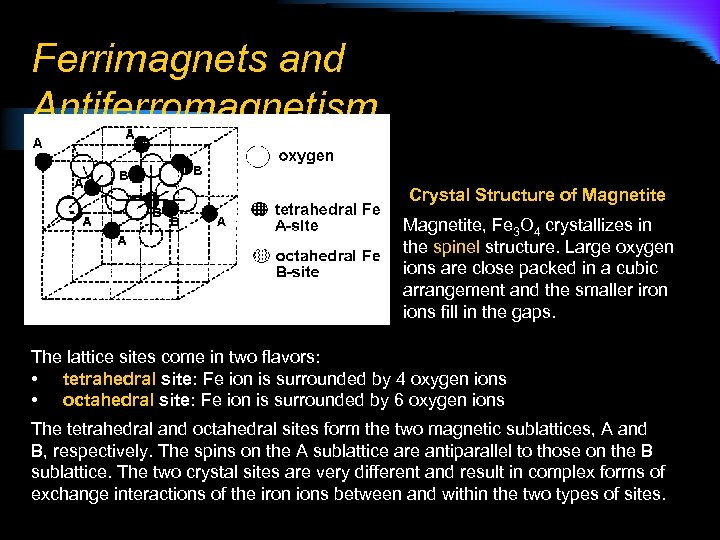Ferrimagnets and Antiferromagnetism Crystal Structure of Magnetite, Fe 3 O 4 crystallizes in the spinel structure. Large oxygen ions are close packed in a cubic arrangement and the smaller iron ions fill in the gaps. The lattice sites come in two flavors: • tetrahedral site: Fe ion is surrounded by 4 oxygen ions • octahedral site: Fe ion is surrounded by 6 oxygen ions The tetrahedral and octahedral sites form the two magnetic sublattices, A and B, respectively. The spins on the A sublattice are antiparallel to those on the B sublattice. The two crystal sites are very different and result in complex forms of exchange interactions of the iron ions between and within the two types of sites.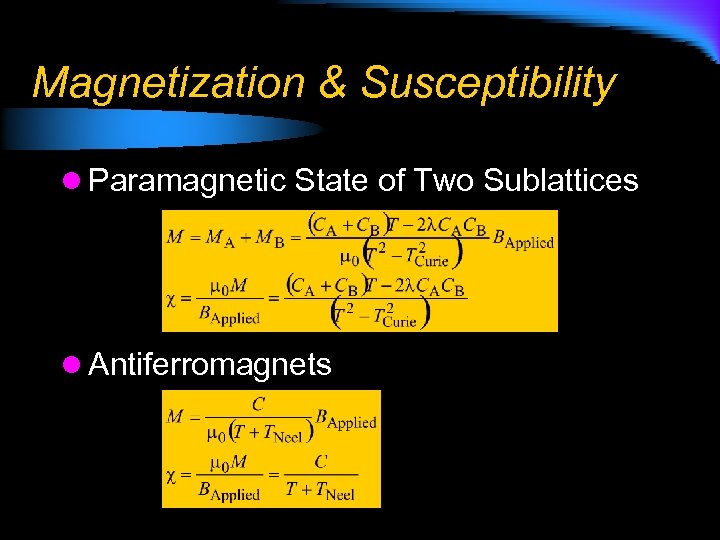Magnetization & Susceptibility l Paramagnetic State of Two Sublattices l Antiferromagnets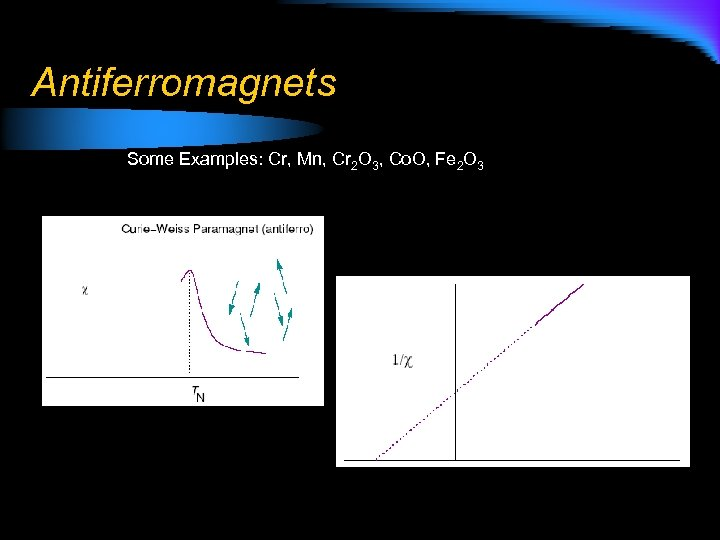Antiferromagnets Some Examples: Cr, Mn, Cr 2 O 3, Co. O, Fe 2 O 3